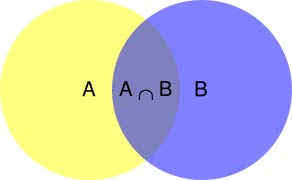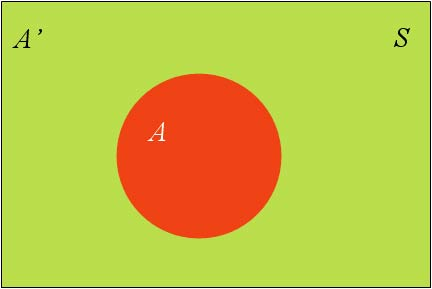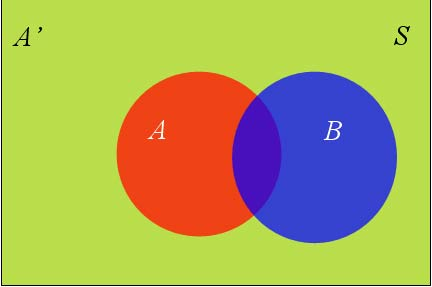# 贝叶斯定理∵ P(A|B) = P(A∩B)/P(B)
∴ P(A∩B) = P(A|B)P(B)

P(A∩B) = P(B|A)P(A)
∴ P(A|B)P(B) = P(B|A)P(A)

P(A|B) = P(B|A)P(A)/P(B)


# 全概率公式P(B) = P(B∩A) +P(B∩A')
∵ P(B∩A) = P(B|A)P(A)
∴ P(B) = P(B|A)P(A) + P(B|A')P(A')# 贝叶斯推断的含义

P(A|B) = P(A)·P(B|A)/P(B)


P(A|B) 称为”后验概率”（Posterior probability），即在B事件发生之后，我们对A事件概率的重新评估。

P(B|A)/P(B) 称为”可能性函数”（Likelyhood），这是一个调整因子，使得预估概率更接近真实概率。

后验概率　＝　先验概率 ｘ 调整因子


# 例子-01-水果糖

## 分析

我们假定，H1表示一号碗，H2表示二号碗。由于这两个碗是一样的，所以P(H1)=P(H2)，也就是说，在取出水果糖之前，这两个碗被选中的概率相同。

P(H1|E) = P(H1)·P(E|H1)/P(E)

P(E) = P(E|H1)P(H1) + P(E|H2)P(H2)

∴ P(E) = 0.75×0.5 + 0.5×0.5 = 0.625;

P(H1|E) = 0.5×0.75/0.625 = 0.6


# 什么是贝叶斯过滤器？

2002年，Paul Graham提出使用”贝叶斯推断”过滤垃圾邮件。他说，这样做的效果，好得不可思议。1000封垃圾邮件可以过滤掉995封，且没有一个误判。

# 建立历史资料库

“训练”过程很简单。首先，解析所有邮件，提取每一个词。然后，计算每个词语在正常邮件和垃圾邮件中的出现频率。比如，我们假定”sex”这个词，在4000封垃圾邮件中，有200封包含这个词，那么它的出现频率就是5%；而在4000封正常邮件中，只有2封包含这个词，那么出现频率就是0.05%。（【注释】如果某个词只出现在垃圾邮件中，Paul Graham就假定，它在正常邮件的出现频率是1%，反之亦然。这样做是为了避免概率为0。随着邮件数量的增加，计算结果会自动调整。）

# 贝叶斯过滤器的使用过程

P(S|W) = P(W|S)P(S) / P(W|S)P(S) + P(W|H)P(H)

 公式中，P(W S)和P(W H)的含义是，这个词语在垃圾邮件和正常邮件中，分别出现的概率。这两个值可以从历史资料库中得到，对sex这个词来说，上文假定它们分别等于5%和0.05%。另外，P(S)和P(H)的值，前面说过都等于50%。

P(S|W) = 5%×50% / 5%×50% + 0.05%×50% = 99%


# 联合概率的计算

 Paul Graham的做法是，选出这封信中 P(S|W) 最高的15个词，计算它们的联合概率。（【注释】如果有的词是第一次出现，无法计算P(S W)，Paul Graham就假定这个值等于0.4。因为垃圾邮件用的往往都是某些固定的词语，所以如果你从来没见过某个词，它多半是一个正常的词。）

# 最终的计算公式